# Net Present Value calculation in Excel (NPV Function)

August 9, 2022
1.8K Views
0

A free Office suite fully compatible with Microsoft OfficeExcel can be used for different sorts of calculations and statistical analysis as well. Sometimes while doing statistical analysis you require to calculate Net present value. Manual calculations of determining the Net present value are complex and laborious. However excel provides a simple solution to this problem i.e. formula of Net Present value which performs Excel Net present Value Calculation WPS . Using this simple formula you can calculate Net present values very easily in your statistical analysis.

This article covers the basic methods to perform Excel Net present Value Calculation WPS  Office (2016/2019/mac/online). Once you go through this article you will be able to easily perform Excel Net present Value Calculation. Let’s cover the simple methodologies of doing Excel Net present Value Calculation WPS Office (2016/2019/mac/online)

Three different ways of performing Excel Net present Value Calculation.

1.1 Using Insert function button in excel to perform Excel Net present Value Calculation

You can perform Excel Net present Value Calculation by using insert function button by following simple below mentioned steps and picture illustrations.

Steps:

1.Open WPS Excel /Spreadsheet file where you want to perform Excel Net present Value Calculation.

2.Click on the cell where you want to perform Excel Net present Value Calculation.

3.Go to Formulas Tab.There will be an Insert formula button in it.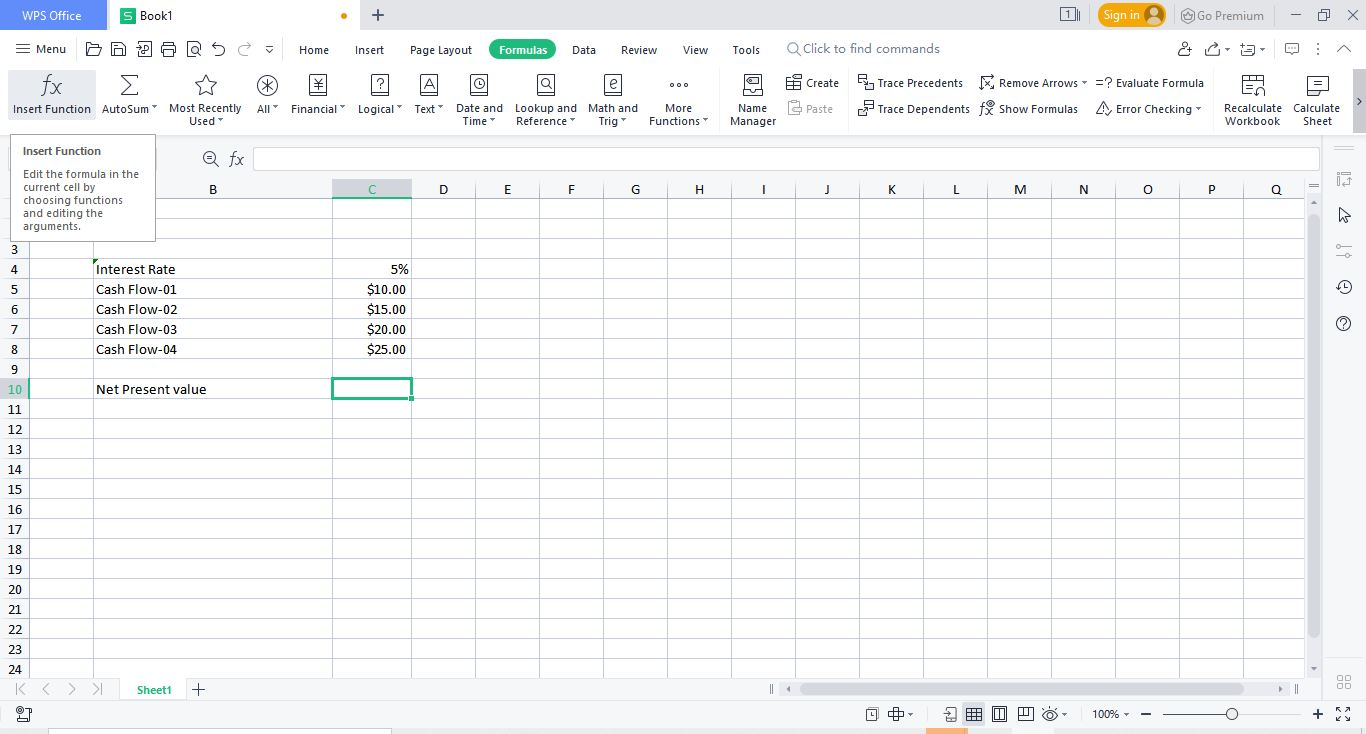4.Click on this button.A new dialogue window shall appear.In the search for a formula bar write “NPV”.NPV (Net present Value) shall appear in the below list.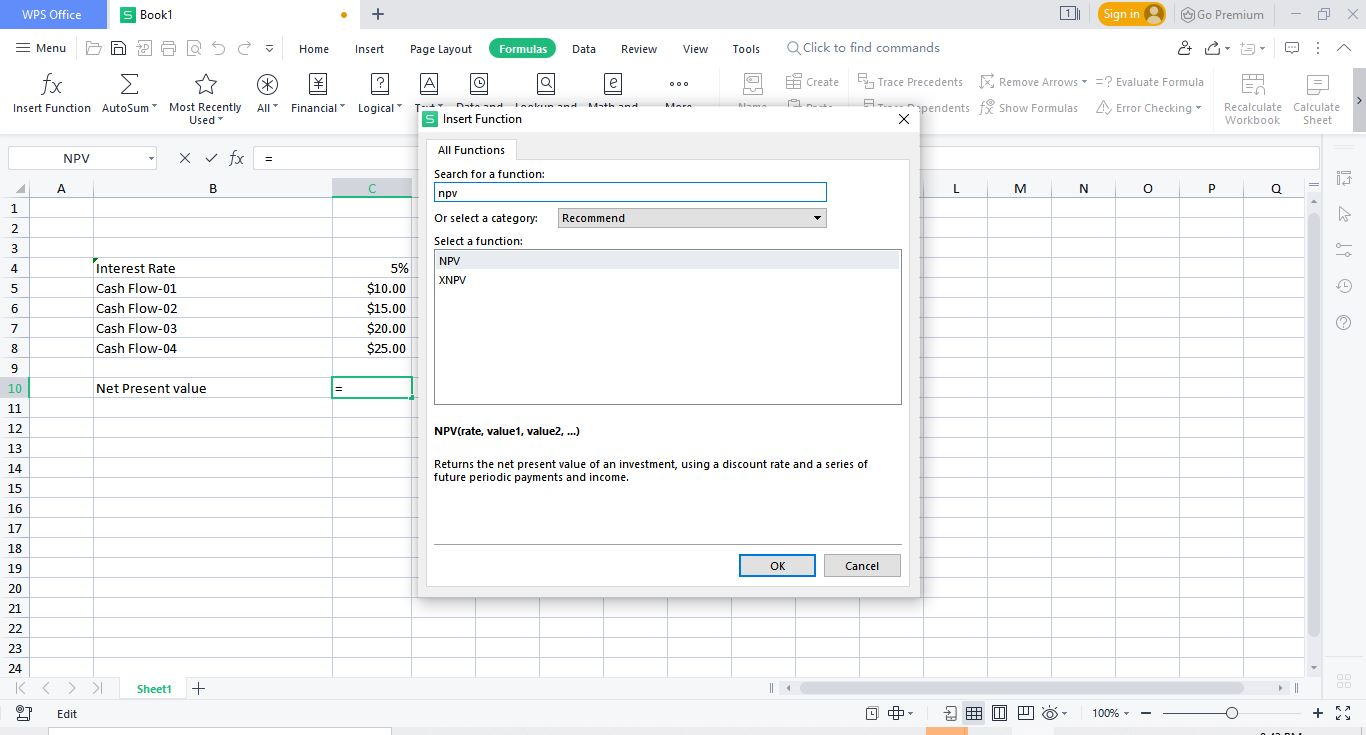5.Click on NPV and press OK.A new function argument window shall appear. To calculate Net present value, two different parameters are required. i.e.Rate (Interest rate per cash flow period I.e. considered as per year) Value (Cash flow(s), for multiple cash flows use “,” in between each period cash flow)

6.You can either enter the values of these two parameters or you can use excel value links as used in picture illustrations in this article.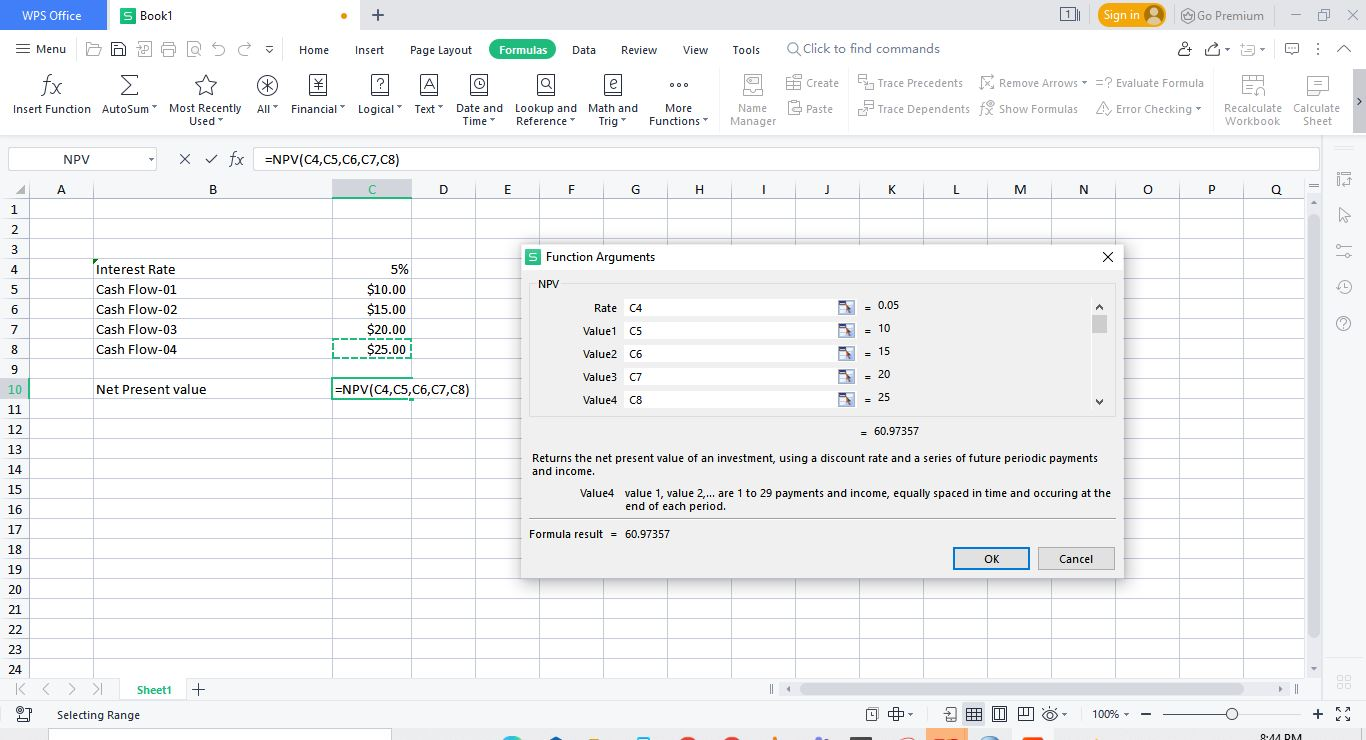7.After entering all two parameters, press enter.Net p data-imgw=800 data-imgh=427 data-src="//res-academy.cache.wpscdn.com/images/seo_posts/20220808/5180405ab0133319a5cd0770abd312e6.png" data-test="skks" style="max-width: 100%; width: 720px; max-height: 384.3px; height: calc(100vw *(427/800)); text-align:center;" >

1.2 Using Financial formula section in excel to perform Excel Net present Value Calculation

You can perform Excel Net present Value Calculation by using financial formula section by following simple below mentioned steps and picture illustrations.

Steps:

1.Open WPS Excel /Spreadsheet file where you want to perform Excel Net present Value Calculation.

2.Click on the cell where you want to perform Excel Net present Value Calculation.

3.Go to Formulas Tab.There will be an financial formula button in it.Click on this button.

4.A drop down list shall appear.In the list, find NPV and click on it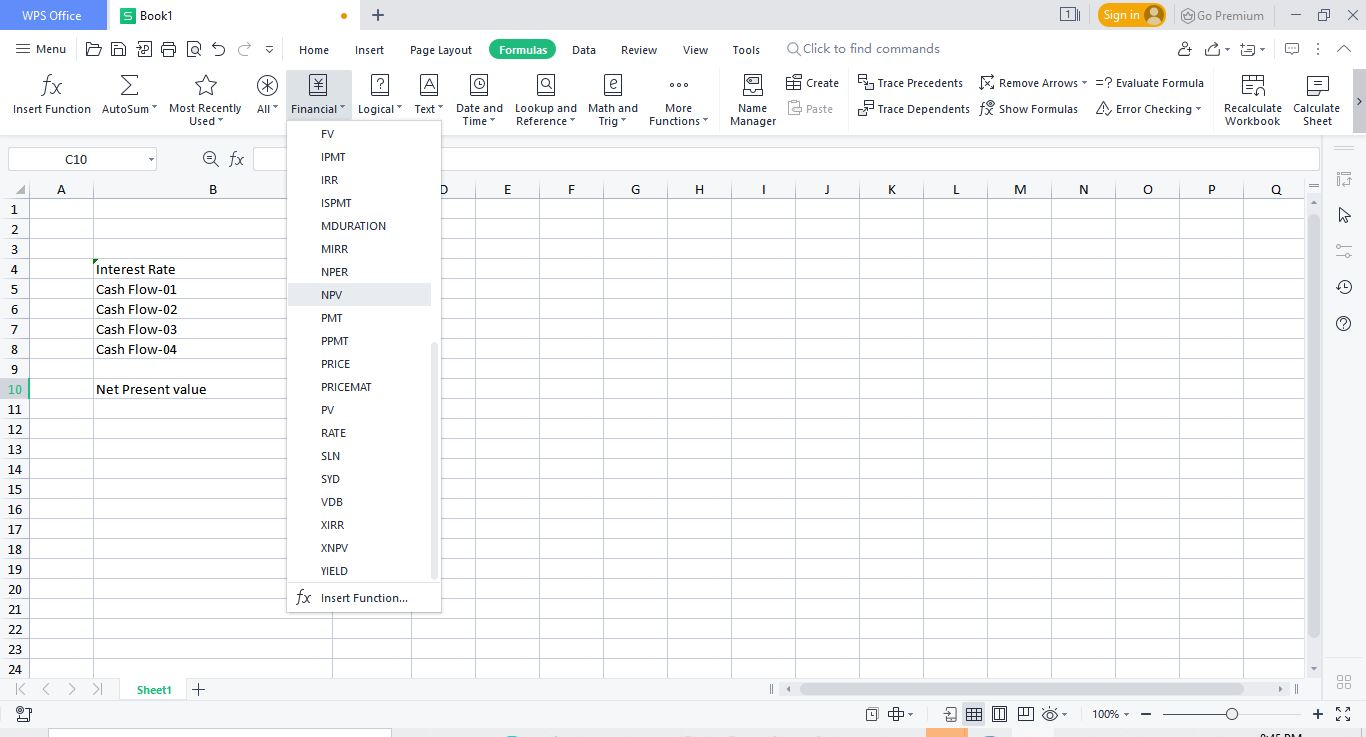5.A new function argument window shall appear. To calculate Net present value, two different parameters are required. i.e.Rate (Interest rate per cash flow period I.e. considered as per year) Value (Cash flow(s), for multiple cash flows use “,” in between each period cash flow)

6.You can either enter the values of these two parameters or you can use excel value links as used in picture illustrations in this article.

7.After entering all two parameters, press enter.Net present value is calculated.

1.3 Manually entering Net present value Formula in excel to perform Excel Net present Value Calculation

You can perform Excel Net present Value Calculation by manually entering Net present value formula by following simple below mentioned steps and picture illustrations.

Steps:

1.Open WPS Excel /Spreadsheet file where you want to perform Excel Net present Value Calculation.

2.Click on the cell where you want to perform Excel Net present Value Calculation.Write “=NPV”, the press Tab.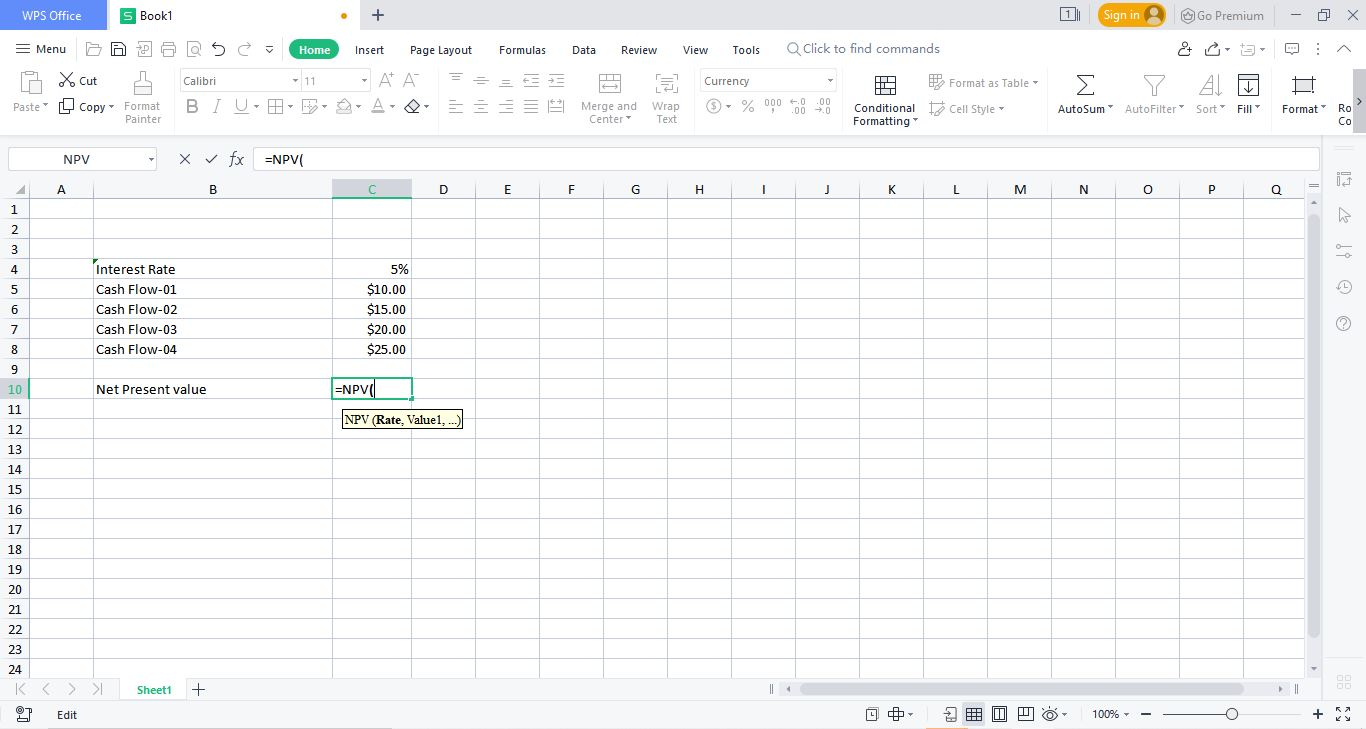3.Net present value formula is initiated.To calculate Net present value, two different parameters are required. i.e.Rate (Interest rate per cash flow period I.e. considered as per year) Value (Cash flow(s), for multiple cash flows use “,” in between each period cash flow)You can either enter the values of these two parameters or you can use excel value links as used in picture illustrations in this article.

4.After entering all two parameters, press enter.

Net present value is calculated.In this way you can easily perform Excel Net present Value Calculation in the WPS excel/spreadsheet.

This article has covered the simplest ways on performing Excel Net present Value Calculationin WPS Excel/Spreadsheet. You can use these simple steps as explained above and easily perform Excel Net present Value Calculation WPS Excel/Spreadsheet whenever required.

Hopefully you have learned How to use perform Excel Net present Value Calculation in WPS Excel/Spreadsheet?  If you want to know more about Excel features, you can follow WPS Academy to learn.